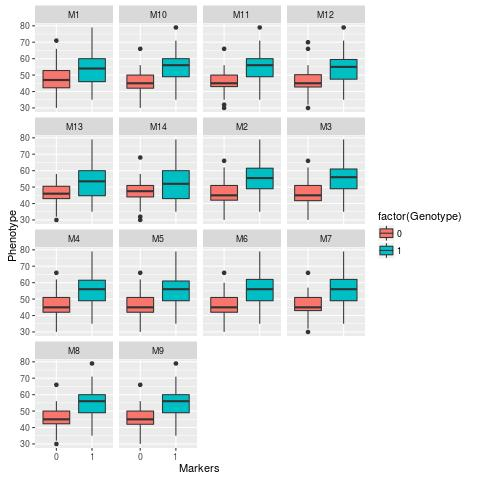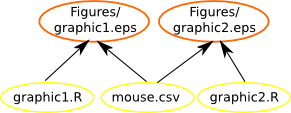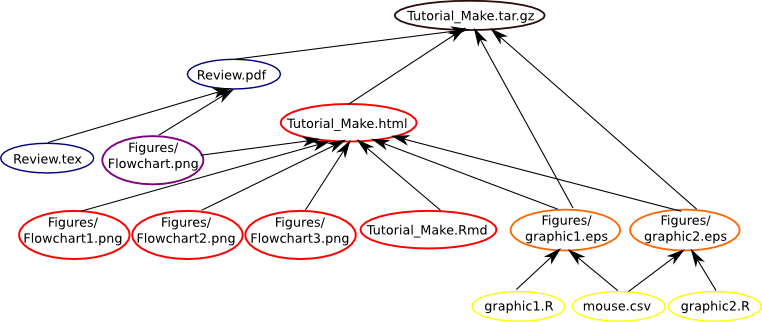ESALQ/USP

# Makefile Tutorial

Written by Cristiane Hayumi Taniguti and Letícia Aparecida de Castro Lara

The goal of this tutorial is to write a simple Makefile to build R graphics, latex and markdown files. This tutorial was developed following the main ideas of the Tutorial Make of the Software Carpentry.

First, we will work with two scripts in R to generate different graphics to analysis of genotypic data of the mouse.cvs file. To obtain these graphics, we must install the packages: ggplot2, dplyr and tidyr. To do this you can open your terminal and follow the commands bellow (considering that you have a Linux system):

For ggplot2:

$wget https://cran.rstudio.com/src/contrib/ggplot2_1.0.1.tar.gz$ R CMD INSTALL ggplot2_1.0.1.tar.gz


For dplyr:

$wget https://cran.rstudio.com/src/contrib/dplyr_0.4.3.tar.gz$ R CMD INSTALL dplyr_0.4.3.tar.gz


For tidyr:

$wget https://cran.rstudio.com/src/contrib/tidyr_0.3.1.tar.gz$ R CMD INSTALL tidyr_0.3.1.tar.gz


### Understanding R files

Let’s run the R script and view the obtained figures.

$Rscript graphic1.R  This script performs a graphic analysis for each of the 14 markers. It can be seen an increase in the phenotypic note with the change of genotype 0 for genotype 1. This first graphic shows the data in histogramsAnother command line that can be used is: $ R CMD BATCH graphic2.R


This other graphics performed by graphic2.R shows the data in box-plots.We can verify that the output of the first command was a .jpeg file and the output of the second control was a .jpeg file and a .Rout file. In the .jpeg files are the graphics generated in each script. The .Rout file is the output of the console.

By choice, we will use the first line since we have no interest in getting the .Rout file. Let’s first sure we start from scratch and delete the .jpeg and .Rout files we created:

$rm *.jpeg *.Rout  ### Our first Makefile Create a file, called Makefile, that will look likes: # Our first Makefile graphic1.jpeg : mouse.csv graphic1.R Rscript graphic1.R  You can read more details about the Make structure at the Review.pdf. By now it is important to know that “graphic1.jpeg” is the target of our rule, it will be build for our action “Rscript graphic1.R” and have the dependencies mouse.csv and graphic1.R. The “#” makes everything that comes after in the line be just a coment. By default, Make looks for a file, called Makefile. The Makefile can have other name. So, if we call it something else we need to tell Make where to find it. For this, we need to use -f flag. $ make

$make -f Makefile2  If show up in the terminal the following message: Makefile:3: *** missing separator. Stop.  it was because we don’t use the TAB character to indent one of our actions. Do it now and try again. We should see in the directory a .jpeg file. If we try re-run our Makefile, we will see: make: 'graphic1.jpeg' is up to date.  This is because our target, graphic1.jpeg, has now been created, and Make will not create it again. Let’s add another rule to the end of Makefile: graphic2.jpeg : mouse.csv graphic2.R Rscript graphic2.R  To run this second command we need to specify the target, because Make attempts to build only the first target it finds in the Makefile, the default target, which is graphic1.jpeg. If we run only Make, then we get: make: 'graphic1.jpeg' is up to date.  So, try to specify the command: $ make graphic2.jpeg


Now, we can see the graphic2.jpeg in the directory.

### Phony target

We may want to remove all our data files so we can explicitly recreate them all. We can introduce a new target in our Makefile, the clean target:

clean :
rm -f *.jpeg


This is an example of a rule that has no dependencies and targets. If we run Make and specify this target,

$make clean  then we get: $ rm -f *.jpeg


If we have a directory called clean,

$make graphic1.jpeg graphic2.jpeg$ mkdir clean
$make clean  then we get: make: 'clean' is up to date.  We can see that we created the .jpeg files again, so why “make clean” didn’t remove them? This is because Make considered that the directory “clean” is the target of the rule and it doesn’t need a update. To avoid this issue we tell Make that this is a phony target, that it does not build anything. This we do by marking the target as .PHONY: .PHONY : clean clean : rm -f *.jpeg  then we get: $ rm -f *.jpeg


We can add a similar command to create all the data files:

.PHONY : all
all : graphic1.jpeg graphic2.jpeg


In this case, we made a rule that the dependencies are targets of other rules. Then, if we run:

$make all  Make will check if the depedecies graphic1.jpeg and graphic2.jpeg are already updated, if isn’t Make will update or create this using the other rules. # Our first Makefile .PHONY : all all : graphic1.jpeg graphic2.jpeg graphic1.jpeg : mouse.csv graphic1.R Rscript graphic1.R graphic2.jpeg : mouse.csv graphic2.R Rscript graphic2.R .PHONY : clean clean : rm -f *.jpeg  By now our Makefile are using the R scripts and the .csv file to build the graphics, as we can see below.### Including .tex and .Rmd file The next step it is to include rules for the .tex file with the goal to build a .pdf file through Latex. The rules will be with the same format that we did for the graphics, we will write the target, dependencies and action. The Review.pdf is a review about Makefile, its building depends of the Review.tex and the figure Figure/Flowchart.png. Our Makefile will include the following rule: Review.pdf: Review.tex Figures/Flowchart.png xelatex Review.tex -bibtex Review.aux xelatex Review.tex xelatex Review.tex rm *.aux *.bbl *.blg *.log  If we include the Review.pdf in .PHONE target and use the make command, we will see the inclusion of the .pdf file in our directory. Don’t forget to add the new target at the actions “all” and “clean”. The construction of our makefile is now given as follows:We can do the same for build this .html tutorial through R Markdown. First, if you don’t have the rmarkdown package follow the commands: $ wget https://cran.rstudio.com/src/contrib/evaluate_0.8.tar.gz
$R CMD INSTALL evaluate_0.8.tar.gz$ wget https://cran.rstudio.com/src/contrib/knitr_1.11.tar.gz
$R CMD INSTALL knitr_1.11.tar.gz$ wget https://cran.rstudio.com/src/contrib/rmarkdown_0.8.1.tar.gz
$R CMD INSTALL rmarkdown_0.8.1.tar.gz  Then, because we want to use the rmarkdown package outside of RStudio, we will need a recent version of pandoc (>= 1.12.3), so verify your version: $ pandoc --version


If yours is older than 1.12.3, you should update it. The way to do it depends of your operational system, you can follow the instruction from here

For us (Ubuntu 14.04 LTS), the commands from Carl Boettiger at this forum works great.

Now, lets create one new rule to build the Tutorial_Make.html from the file Tutorial_Make.Rmd.

Tutorial_Make.html: Tutorial_Make.Rmd Figures/graphic1.jpeg Figures/graphic2.jpeg Figures/Flowchart1.png Figures/Flowchart2.png Figures/Flowchart3.png Figures/Flowchart.png
R -e "rmarkdown::render('Tutorial_Make.Rmd')"


As above, our Makefile is using the R scripts and the .csv to build the graphics, the .tex file and a figure to build the .pdf file and the graphics, three figures and the .Rmd file to build the .html file, as we can see below.Now we want to compress the main files built on our tutorial in a file named Tutorial_Make.tar.gz, so just one more rule.

Tutorial_Make.tar.gz : Figures/graphic1.jpeg Figures/graphic2.jpeg Tutorial_Make.html Review.pdf
tar -czf Tutorial_Make.tar.gz Figures/graphic1.jpeg Figures/graphic2.jpeg Tutorial_Make.html Review.pdf


Now, our Makefile should look like this:

# Our first Makefile
.PHONY : all
all : Figures/graphic1.jpeg Figures/graphic2.jpeg Review.pdf Tutorial_Make.html Tutorial_Make.tar.gz

Figures/graphic1.jpeg : graphic1.R mouse.csv
Rscript graphic1.R
mv graphic1.jpeg Figures

Figures/graphic2.jpeg : graphic2.R mouse.csv
Rscript graphic2.R
mv graphic2.jpeg Figures

Review.pdf: Review.tex Figures/Flowchart.png
xelatex Review.tex
-bibtex Review.aux
xelatex Review.tex
xelatex Review.tex
rm *.aux *.bbl *.blg *.log

Tutorial_Make.html: Tutorial_Make.Rmd Figures/graphic1.jpeg Figures/graphic2.jpeg Figures/Flowchart1.png Figures/Flowchart2.png Figures/Flowchart3.png Figures/Flowchart.png
R -e "rmarkdown::render('Tutorial_Make.Rmd')"

Tutorial_Make.tar.gz : Figures/graphic1.jpeg Figures/graphic2.jpeg Tutorial_Make.html Review.pdf
tar -czf Tutorial_Make.tar.gz Figures/graphic1.jpeg Figures/graphic2.jpeg Tutorial_Make.html Review.pdf

.PHONY : clean
clean :
rm *.pdf *.html *.gz
cd Figures; rm -f *.jpeg


Our final Makefile, therefore, works as follows:### Automatic variables

As we can see, our Makefile have a lot of repetitive terms, with this is more easy to understand what is happening, but once we already know what we are doing we can simplify all of this using some others resources of Make.

One way to simplify and reduce our makefile is with the automatic variables. This allow to remove duplication in the file. The command $@ is used to refer to the target of the current rule, the$^ to refer to the dependencies of the current rule and the $< to refer to the first dependency of the current rule. So, our Makefile also can writing like: # Our second Makefile .PHONY : all all : Figures/graphic1.jpeg Figures/graphic2.jpeg Review.pdf Tutorial_Make.html Tutorial_Make.tar.gz Figures/graphic1.jpeg : graphic1.R mouse.csv Rscript$<
mv graphic1.jpeg Figures

Figures/graphic2.jpeg : graphic2.R mouse.csv
Rscript $< mv graphic2.jpeg Figures Review.pdf : Review.tex Figures/Flowchart.png xelatex$<
-bibtex Review.aux
xelatex $< xelatex$<
rm *.aux *.bbl *.blg *.log

Tutorial_Make.html: Tutorial_Make.Rmd Figures/graphic1.jpeg Figures/graphic2.jpeg Figures/Flowchart.png  Figures/Flowchart1.png  Figures/Flowchart2.png Figures/Flowchart3.png
R -e "rmarkdown::render('$<')" Tutorial_Make.tar.gz : Figures/graphic1.jpeg Figures/graphic2.jpeg Tutorial_Make.html Review.pdf tar -czf$@ $^ .PHONY : clean clean : rm *.pdf *.html *.gz cd Figures; rm -f *.jpeg  It is importante to know that you can’t use the wildcard, “*.jpeg”, to name the dependencies of the target, because Make use it as a file name itself, and there is no file matching that, nor any rule so we get an error. Make have it own wildcards, in our next step we will learn how to use them. As we can see, we have a lot of repeated names in our code, we can substitute them for % in targets and dependecies and$* in actions. You can do this only with the words that match in the same rule.

Applying those informations our makefile will be like this:

# Our second Makefile
.PHONY : all
all : Figures/graphic1.jpeg Figures/graphic2.jpeg Review.pdf Tutorial_Make.html Tutorial_Make.tar.gz

Figures/%.jpeg : %.R mouse.csv
Rscript $< mv *.jpeg Figures %.pdf : %.tex Figures/Flowchart.png xelatex$<
-bibtex $*.aux xelatex$<
xelatex $< rm *.aux *.bbl *.blg *.log %.html: %.Rmd Figures/graphic1.jpeg Figures/graphic2.jpeg Figures/Flowchart.png Figures/Flowchart1.png Figures/Flowchart2.png Figures/Flowchart3.png R -e "rmarkdown::render('$<')"

%.tar.gz : Figures/graphic1.jpeg Figures/graphic2.jpeg %.html Review.pdf
tar -czf $@$^

.PHONY : clean
clean :
rm *.pdf *.html *.gz
cd Figures; rm -f *.jpeg


### Variables

Despite replace some names for automatic variables, our Makefile still have repeated content. If necessary modify it, we would have to upgrade our Makefile in several places. Thus, it becomes interest to assign variables for repeated terms and create functions, facilitating the keeping of the script.

Let’s create the variables: FIG1_JPEG and FIG2_JPEG

# Our second Makefile

FIG1_JPEG=Figures/graphic1.jpeg
FIG2_JPEG=Figures/graphic2.jpeg
REV_PDF=Review.pdf

.PHONY : all
all : $(FIG1_JPEG)$(FIG2_JPEG) $(REV_PDF) Tutorial_Make.html Tutorial_Make.tar.gz Figures/%.jpeg : %.R mouse.csv Rscript$<
mv *.jpeg Figures

%.pdf : %.tex Figures/Flowchart.png
xelatex $< -bibtex$*.aux
xelatex $< xelatex$<
rm *.aux *.bbl *.blg *.log

%.html: %.Rmd $(FIG1_JPEG)$(FIG2_JPEG) Figures/Flowchart.png  Figures/Flowchart1.png  Figures/Flowchart2.png  Figures/Flowchart3.png
R -e "rmarkdown::render('$<')" %.tar.gz :$(FIG1_JPEG) $(FIG2_JPEG) %.html$(REV_PDF)
tar -czf $@$^

.PHONY : clean
clean :
rm *.pdf *.html *.gz
cd Figures; rm -f *.jpeg


The variables use to come at the top of the Makefile, because it is easier to find and modify them. Another option is to create a new file that contains only the definitions of the variables and then we call this file in our Makefile. In this way, we will create the var.mk file:

FIG1_JPEG=Figures/graphic1.jpeg
FIG2_JPEG=Figures/graphic2.jpeg
REV_PDF=Review.pdf


We can then import this Makefile into Makefile using:

include var.mk


Then, our Makefile looks like:

# Our second Makefile

include var.mk

.PHONY : all
all : $(FIG1_JPEG)$(FIG2_JPEG) $(REV_PDF) Tutorial_Make.html Tutorial_Make.tar.gz Figures/%.jpeg : %.R mouse.csv Rscript$<
mv *.jpeg Figures

%.pdf : %.tex Figures/Flowchart.png
xelatex $< -bibtex$*.aux
xelatex $< xelatex$<
rm *.aux *.bbl *.blg *.log

%.html: %.Rmd $(FIG1_JPEG)$(FIG2_JPEG) Figures/Flowchart.png  Figures/Flowchart1.png  Figures/Flowchart2.png  Figures/Flowchart3.png
R -e "rmarkdown::render('$<')" %.tar.gz :$(FIG1_JPEG) $(FIG2_JPEG) %.html$(REV_PDF)
tar -czf $@$^

.PHONY : clean
clean :
rm *.pdf *.html *.gz
cd Figures; rm -f *.jpeg


We can re-run Make to see that everything still works:

$make clean$ make


### Functions

There are two useful functions of Make, which are:

Make’s wildcard function: to get lists of files matching a pattern.

Make’s patsubst function: to rewrite file names.

Make has many functions which can be used to write more complex rules. The wildcard function gets a list of files matching some pattern, which we can then save in a variable. So, for example, we can get a list of all our .png files and save these in a variable by adding this at the beginning of our makefile:

PNG_FILES=$(wildcard Figures/*.png)  The patsubst function takes a pattern, a replacement string and a list of names in that order; each name in the list that matches the pattern is replaced by the replacement string. Again, we can save the result in a variable. So, for example, we can rewrite our list of .R files into a list of data files (files ending in .jpeg) and save these in a variable. For this, we will define the list of .R files using the previous function. R_FILES=$(wildcard *.R)
JPEG_FILES=$(patsubst %.R, %.jpeg,$(R_FILES))


Then, our Makefile looks like:

# Our second Makefile

REV_PDF=Review.pdf
PNG_FILES=$(wildcard Figures/*.png) R_FILES=$(wildcard *.R)
JPEG_FILES=$(patsubst %.R, Figures/%.jpeg,$(R_FILES))

.PHONY : all
all : $(JPEG_FILES)$(REV_PDF) Tutorial_Make.html Tutorial_Make.tar.gz

Figures/%.jpeg : %.R mouse.csv
Rscript $< mv *.jpeg Figures %.pdf : %.tex Figures/Flowchart.png xelatex$<
-bibtex $*.aux xelatex$<
xelatex $< rm *.aux *.bbl *.blg *.log %.html: %.Rmd$(JPEG_FILES) $(PNG_FILES) R -e "rmarkdown::render('$<')"

%.tar.gz : $(JPEG_FILES) %.html$(REV_PDF)
tar -czf $@$^

.PHONY : clean
clean :
rm *.pdf *.html *.gz
cd Figures; rm -f $(JPEG_FILES)  Like to the previous variables, for functions also is possible use the var.mk file in our Makefile. However, the var.mk now looks like: REV_PDF=Review.pdf PNG_FILES=$(wildcard Figures/*.png)
R_FILES=$(wildcard *.R) JPEG_FILES=$(patsubst %.R, Figures/%.jpeg, \$(R_FILES))